### 2.2.3.2 Magnetostatic potential. The DADI method

The micromagnetism poses particular emphasis in the exact calculation of the demagnetizing field. In the continuous micromagnetic approach it is described by the Maxwell equation in the absence of currentsand, therefore, there exists a potential called scalar magnetostatic potential that satisfies: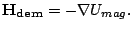(2.26)

This potential satisfies the Laplace equation outside the material and the Poisson one inside the material: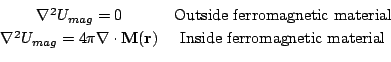(2.27)

with the boundary conditions: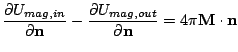(2.28)

where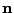is the unit normal to surface of the ferromagnetic sample.

The sources of the magnetostatic field are called magnetostatic charges. The surface charge is defined asand the volume charge as. The magnetostatic energy integral extends to all the space, not only the ferromagnetic body, and the minimization of the magnetostatic energy will seek the reduction of the field outside the ferromagnetic body. This can be achieved reducing of the magnetic charges and that fact is stated as the pole avoidance principle. This field gives origin to domains and magnetization inhomogeneities. The generic solution of a field from its sources is found in traditional electromagnetism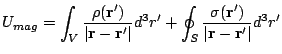(2.29)

and from Eq. (2.26)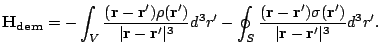(2.30)

In micromagnetic calculations most of the computation time is spent in the calculation of the magnetostatic field. The dipolar interaction is non-local and long ranged. The field in dipole-dipole representation is a sum of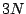elements, where N is the number of atomic moments and the numerical integration of Eq. (2.30) scales likeand this represents too much computational time even for today advanced computers. Due to this reason, several advanced methods have been used to solve the magnetostatic problem. The methods used are Fast Fourier Transform(FFT) [Yuan 92], Finite Element Method (FEM) [Chen 97], hybrid FEM - Boundary Element Method (FEM-BEM) [Fredkin 90], Fast Multipole Method (FMM) [Brown 04] and other advanced methods. The efficiency of each method depends on the implementation and on the simulated physical problem. We use a different method called the Dynamic Alternating Direction Implicit (DADI) [Gibbons 98]. The underlying principle of this method is to add a fictitious time derivative to Eq. (2.27):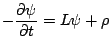(2.31)

with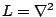. Next, we integrate the resulting equation forward in time to the time-asymptotic state. At this point the fictitious time derivative goes to zero and we have the desired solution. The ideal case would be the performance of only one step of integration of Eq. (2.31). The time stepshould be chosen to achieve the convergence in as few steps as possible. In a hysteresis loop the solution is obtained from the solution of the previous field step, thus the test potential is very close to the solution and the method converges in few steps. Near coercivity large changes of magnetization and scalar potential are expected. In this situation, the complete convergence is needed.

The operatoris replaced by its finite difference equivalent. For example the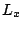contribution is(2.32)

with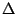- the size of the spatial discretization andthe value of the scalar potential in the element on the node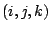. The method consists of three passes(2.33)

The magnetostatic volume charges are included replacing the operator divergence by its finite difference equivalent. In the surface cells we have to change this term by the surface charges. For example the contribution of a cell which occupy the position (i,j,k) will be: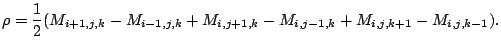(2.34)

The solution of these equations involve tridiagonal matrices, which are linear systems easy to solve. This can be easily seen from Eq. (2.32) because the left hand side only includes the values of,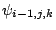and. The evaluation of the potential is the successive solution of Eqs. (2.33). From the current value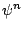, we obtain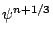, and this yields the value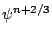and, finally, the value of the potential in the next step.

This method needs the addition of free (zero magnetization) space in the simulated region to allow the potential to slowly tend to its infinity value. This value is arbitrary because the equation is differential and we choose it, for convenience, to be zero. This is also the case in FEM and FFT methods(zero-padding). The hybrid FEM-BEM method presents the advantage of not requiring discretization elements outside the simulated sample. In the DADI method periodic boundary is easily implemented if we consider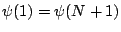. The resulting tridiagonal system is replaced then by a periodic tridiagonal system, which is also very simple to solve.

2008-04-04